# matplotlib.axes.Axes.hist#

Axes.hist(x, bins=None, range=None, density=False, weights=None, cumulative=False, bottom=None, histtype='bar', align='mid', orientation='vertical', rwidth=None, log=False, color=None, label=None, stacked=False, *, data=None, **kwargs)[source]#

Compute and plot a histogram.

This method uses `numpy.histogram` to bin the data in x and count the number of values in each bin, then draws the distribution either as a `BarContainer` or `Polygon`. The bins, range, density, and weights parameters are forwarded to `numpy.histogram`.

If the data has already been binned and counted, use `bar` or `stairs` to plot the distribution:

```counts, bins = np.histogram(x)
plt.stairs(counts, bins)
```

Alternatively, plot pre-computed bins and counts using `hist()` by treating each bin as a single point with a weight equal to its count:

```plt.hist(bins[:-1], bins, weights=counts)
```

The data input x can be a singular array, a list of datasets of potentially different lengths ([x0, x1, ...]), or a 2D ndarray in which each column is a dataset. Note that the ndarray form is transposed relative to the list form. If the input is an array, then the return value is a tuple (n, bins, patches); if the input is a sequence of arrays, then the return value is a tuple ([n0, n1, ...], bins, [patches0, patches1, ...]).

Parameters:
x(n,) array or sequence of (n,) arrays

Input values, this takes either a single array or a sequence of arrays which are not required to be of the same length.

binsint or sequence or str, default: `rcParams["hist.bins"]` (default: `10`)

If bins is an integer, it defines the number of equal-width bins in the range.

If bins is a sequence, it defines the bin edges, including the left edge of the first bin and the right edge of the last bin; in this case, bins may be unequally spaced. All but the last (righthand-most) bin is half-open. In other words, if bins is:

```[1, 2, 3, 4]
```

then the first bin is `[1, 2)` (including 1, but excluding 2) and the second `[2, 3)`. The last bin, however, is `[3, 4]`, which includes 4.

If bins is a string, it is one of the binning strategies supported by `numpy.histogram_bin_edges`: 'auto', 'fd', 'doane', 'scott', 'stone', 'rice', 'sturges', or 'sqrt'.

rangetuple or None, default: None

The lower and upper range of the bins. Lower and upper outliers are ignored. If not provided, range is `(x.min(), x.max())`. Range has no effect if bins is a sequence.

If bins is a sequence or range is specified, autoscaling is based on the specified bin range instead of the range of x.

densitybool, default: False

If `True`, draw and return a probability density: each bin will display the bin's raw count divided by the total number of counts and the bin width (`density = counts / (sum(counts) * np.diff(bins))`), so that the area under the histogram integrates to 1 (`np.sum(density * np.diff(bins)) == 1`).

If stacked is also `True`, the sum of the histograms is normalized to 1.

weights(n,) array-like or None, default: None

An array of weights, of the same shape as x. Each value in x only contributes its associated weight towards the bin count (instead of 1). If density is `True`, the weights are normalized, so that the integral of the density over the range remains 1.

cumulativebool or -1, default: False

If `True`, then a histogram is computed where each bin gives the counts in that bin plus all bins for smaller values. The last bin gives the total number of datapoints.

If density is also `True` then the histogram is normalized such that the last bin equals 1.

If cumulative is a number less than 0 (e.g., -1), the direction of accumulation is reversed. In this case, if density is also `True`, then the histogram is normalized such that the first bin equals 1.

bottomarray-like, scalar, or None, default: None

Location of the bottom of each bin, i.e. bins are drawn from `bottom` to `bottom + hist(x, bins)` If a scalar, the bottom of each bin is shifted by the same amount. If an array, each bin is shifted independently and the length of bottom must match the number of bins. If None, defaults to 0.

histtype{'bar', 'barstacked', 'step', 'stepfilled'}, default: 'bar'

The type of histogram to draw.

• 'bar' is a traditional bar-type histogram. If multiple data are given the bars are arranged side by side.

• 'barstacked' is a bar-type histogram where multiple data are stacked on top of each other.

• 'step' generates a lineplot that is by default unfilled.

• 'stepfilled' generates a lineplot that is by default filled.

align{'left', 'mid', 'right'}, default: 'mid'

The horizontal alignment of the histogram bars.

• 'left': bars are centered on the left bin edges.

• 'mid': bars are centered between the bin edges.

• 'right': bars are centered on the right bin edges.

orientation{'vertical', 'horizontal'}, default: 'vertical'

If 'horizontal', `barh` will be used for bar-type histograms and the bottom kwarg will be the left edges.

rwidthfloat or None, default: None

The relative width of the bars as a fraction of the bin width. If `None`, automatically compute the width.

Ignored if histtype is 'step' or 'stepfilled'.

logbool, default: False

If `True`, the histogram axis will be set to a log scale.

colorcolor or array-like of colors or None, default: None

Color or sequence of colors, one per dataset. Default (`None`) uses the standard line color sequence.

labelstr or None, default: None

String, or sequence of strings to match multiple datasets. Bar charts yield multiple patches per dataset, but only the first gets the label, so that `legend` will work as expected.

stackedbool, default: False

If `True`, multiple data are stacked on top of each other If `False` multiple data are arranged side by side if histtype is 'bar' or on top of each other if histtype is 'step'

Returns:
narray or list of arrays

The values of the histogram bins. See density and weights for a description of the possible semantics. If input x is an array, then this is an array of length nbins. If input is a sequence of arrays `[data1, data2, ...]`, then this is a list of arrays with the values of the histograms for each of the arrays in the same order. The dtype of the array n (or of its element arrays) will always be float even if no weighting or normalization is used.

binsarray

The edges of the bins. Length nbins + 1 (nbins left edges and right edge of last bin). Always a single array even when multiple data sets are passed in.

patches`BarContainer` or list of a single `Polygon` or list of such objects

Container of individual artists used to create the histogram or list of such containers if there are multiple input datasets.

Other Parameters:
dataindexable object, optional

If given, the following parameters also accept a string `s`, which is interpreted as `data[s]` (unless this raises an exception):

x, weights

**kwargs

`Patch` properties

`hist2d`

2D histogram with rectangular bins

`hexbin`

2D histogram with hexagonal bins

Notes

For large numbers of bins (>1000), plotting can be significantly faster if histtype is set to 'step' or 'stepfilled' rather than 'bar' or 'barstacked'.

## Examples using `matplotlib.axes.Axes.hist`#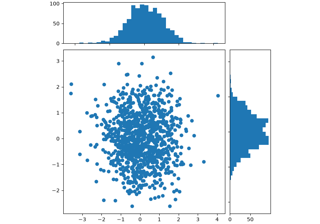Scatter plot with histograms

Scatter plot with histograms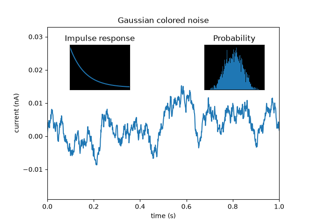Axes Demo

Axes Demo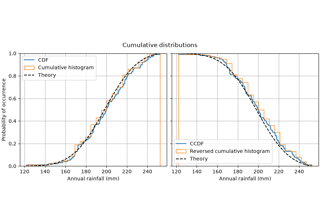Using histograms to plot a cumulative distribution

Using histograms to plot a cumulative distributionSome features of the histogram (hist) function

Some features of the histogram (hist) function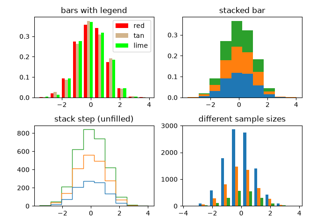The histogram (hist) function with multiple data sets

The histogram (hist) function with multiple data sets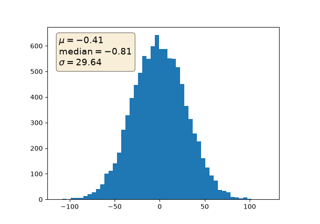Placing text boxes

Placing text boxes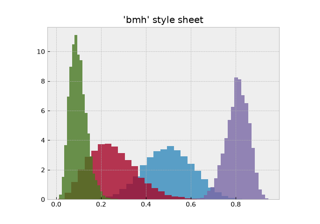Bayesian Methods for Hackers style sheet

Bayesian Methods for Hackers style sheet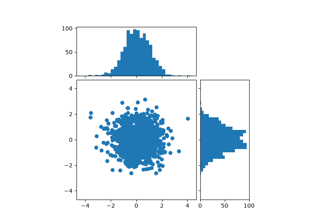Scatter Histogram (Locatable Axes)

Scatter Histogram (Locatable Axes)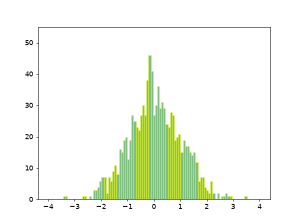Animated histogram

Animated histogram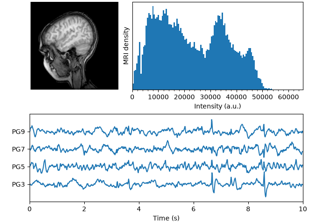MRI with EEG

MRI with EEG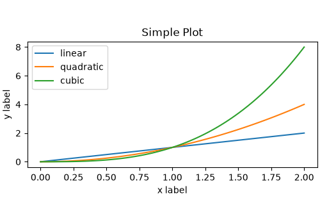Quick start guide

Quick start guide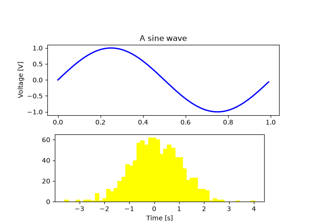Artist tutorial

Artist tutorialPath Tutorial

Path Tutorial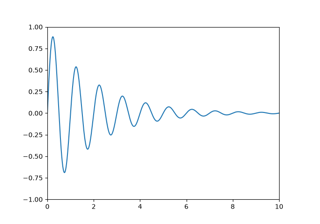Transformations Tutorial

Transformations Tutorial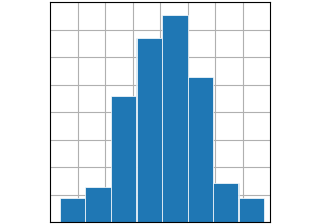hist(x)

hist(x)